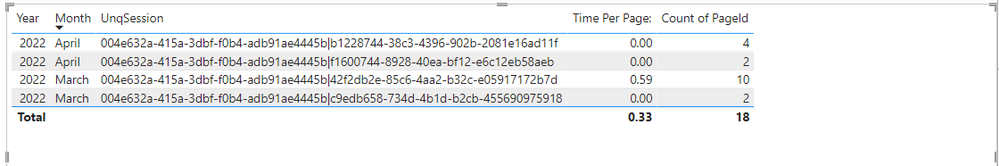cancel
Showing results for
Did you mean:Helper I

## Sum pages visited per session

Alright.... So I want to create a measure that will sum up the number of pages visited per session.

I want to sum the count of the URL column for each UnqSession, and have the ability to average it over time or industry (etc)I can lay it out in PBI Table and it looks like this, but I need measures so I can look at averages by month, etc..2 ACCEPTED SOLUTIONSSuper User

Count till Date avg above that

AverageX(Values(Table[Date]), Calculate(Distinctcount(Table[PageID])))

Count till Month avg above that

AverageX(Values(Table[Month Year]), Calculate(Distinctcount(Table[PageID])))

you can use count in place of DistinctcountSuper User

Hi,

In the Query Editor, seperate the Date and time stamp in 2 columns from the Eventtime column.  Create a Calendar Table with calculated column formulas  for Year, Month name and Month number.  Sort the Month name by the Month number.  Create a relatinoship (Many to One and Single) between the Event Date column and Date column of the Calendar Table.  To your visual, drag Year and Month name from the Calendar Table.

Write this measure

Measure = counta(Data[URL])

Hope this helps.

Regards,
Ashish Mathur
http://www.ashishmathur.com
3 REPLIES 3Helper I

Thank y'all so so muchSuper User

Hi,

In the Query Editor, seperate the Date and time stamp in 2 columns from the Eventtime column.  Create a Calendar Table with calculated column formulas  for Year, Month name and Month number.  Sort the Month name by the Month number.  Create a relatinoship (Many to One and Single) between the Event Date column and Date column of the Calendar Table.  To your visual, drag Year and Month name from the Calendar Table.

Write this measure

Measure = counta(Data[URL])

Hope this helps.

Regards,
Ashish Mathur
http://www.ashishmathur.comSuper User

Count till Date avg above that

AverageX(Values(Table[Date]), Calculate(Distinctcount(Table[PageID])))

Count till Month avg above that

AverageX(Values(Table[Month Year]), Calculate(Distinctcount(Table[PageID])))

you can use count in place of Distinctcount